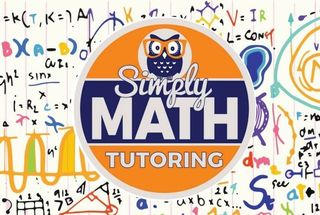\$849.00 - \$849.00

# 8th Grade – 4 week Math BootCamp• Ages:

Operations with integers

®    Integer addition and subtraction rules

®    Add and subtract integers using counters

®    Add and subtract three or more integers

Rational numbers

®    Identify rational and irrational numbers

®    Write fractions in lowest terms

®    Lowest common denominator

®    Round decimals and mixed numbers

Operations with rational numbers

Reciprocals and multiplicative inverses
Add and subtract rational numbers: word problems
Multiply and divide rational numbers
Exponents and roots

Understanding exponents
Evaluate exponents
Solve equations with variable exponents
Exponents with negative bases
Exponents with decimal and fractional bases
Understanding negative exponents
Evaluate negative exponents
Multiplication with exponents
Division with exponents
Proportional relationships

Find the constant of proportionality from a table
Write equations for proportional relationships from tables
Identify proportional relationships by graphing
Find the constant of proportionality from a graph
Coordinate plane

Points on a coordinate plane
Follow directions on a coordinate plane
Two-dimensional figures

Identify and classify polygons
Classify triangles
Identify trapeziums
Properties of parallelograms
Properties of rhombuses
Properties of squares and rectangles
Congruence and similarity

Similar and congruent figures
Side lengths and angle measures of congruent figures
Congruence statements and corresponding parts
Congruent triangles: SSS, SAS and ASA
Pythagoras’ theorem

Pythagoras’ theorem: find the length of the hypotenuse
Pythagoras’ theorem: find the missing leg length
Pythagoras’ theorem: find the perimeter
Probability

Probability of simple events
Probability of opposite, mutually exclusive and overlapping events
Experimental probability
Make predictions
Compound events: find the number of outcomes